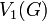# Equal marginal subgroups for a subvariety not implies equal verbal subgroups

## Statement

It is possible to have a group$G$ and two subvarieties$\mathcal{V}_1$ and$\mathcal{V}_2$ of the variety of groups such that the following hold:

•$V_1(G) \ne V_2(G)$, where$V_1(G)$ and$V_2(G)$ denote respectively the$\mathcal{V}_1$-verbal subgroup and$\mathcal{V}_2$-verbal subgroup of$G$.
•$V_1^*(G) = V_2^*(G)$, where$V_1^*(G)$ and$V_2^*(G)$ denote respectively the$\mathcal{V}_1$-marginal subgroup and$\mathcal{V}_2$-marginal subgroup of$G$.

## Proof

### Perfect and centerless

Consider an example where:

•$G$ is a centerless group that is not a perfect group (see centerless not implies perfect), for instance,$G$ is isomorphic to symmetric group:S3.
•$\mathcal{V}_1$ is the variety of abelian groups.
•$\mathcal{V}_2$ is the variety comprising the trivial group.

Then:

•$V_1(G) = [G,G]$ is the derived subgroup of$G$. Since$G$ is not perfect,$V_1(G) \ne G$.
•$V_2(G) = G$.
• Thus,$V_1(G) \ne V_2(G)$.

However:

•$V_1^*(G)$ is the center of$G$, which is trivial since$G$ is centerless.
•$V_2^*(G)$ is the trivial subgroup of$G$.
• Thus,$V_1^*(G) = V_2^*(G)$.

### Example with a finite nilpotent group

Consider the example where:

•$G$ is the nontrivial semidirect product of Z4 and Z4.
•$\mathcal{V}_1$ is the variety of abelian groups.
•$\mathcal{V}_2$ is the variety of groups of exponent two (along with the trivial group).

Then:

•$V_1(G)$ is derived subgroup of nontrivial semidirect product of Z4 and Z4. In particular, is the derived subgroup of$G$, and is isomorphic to cyclic group:Z2.
•$V_2(G)$ is the Frattini subgroup of$G$, and it coincides with the center of nontrivial semidirect product of Z4 and Z4, which is isomorphic to Klein four-group.
•$V_1^*(G)$ is the center of$G$, i.e., it is the center of nontrivial semidirect product of Z4 and Z4, and it is isomorphic to the Klein four-group.
•$V_2^*(G) = \Omega_1(Z(G))$ is the socle of$G$ (see socle equals Omega-1 of center in nilpotent p-group), and it in fact coincides with the center, since the center is an elementary abelian group.